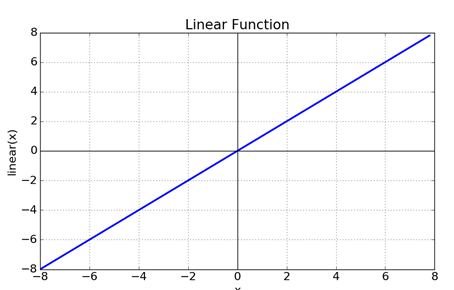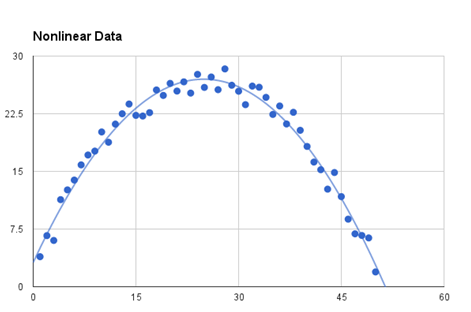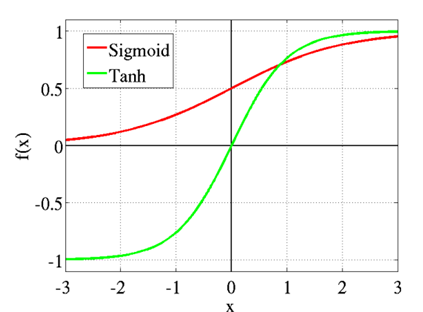/  Technology   /  Activation Functions in Neural Networks## Activation Functions in Neural Networks

Activation function is used to decide, whether a neuron should be activated or not. It is decided by calculating weighted sum and further adding bias with it.It helps to determine the output of neural network like yes or no. It gives the resulting values in between 0 to 1 or -1 to 1 etc.

The Activation Function is broadly divided into 2 types-

1. Linear Activation Function
2. Non-linear Activation Functions

#### Linear or Identity Activation FunctionAs you can see above, the output of the functions will not be confined between any range.

Equation :  f(x) = x

Range : (-infinity to infinity)

It doesn’t work with the complex or various parameters of usual data that is fed to the neural networks.

#### Non-linear Activation FunctionIt makes non-linear transformation on the input and adapts with variety of data and to differentiate between the output.

The Nonlinear Activation Functions are the widely used activation functions.

The Nonlinear Activation Functions are primarily divided on the basis of their range or curves

### 1. Sigmoid or Logistic Activation Function

The Sigmoid Function curve looks like a S-shape.• Equation : A = 1/(1 + e-x)
• Nature :Non-linear. Notice that z values lies between -2 to 2, Y values are very steep. This means, small changes in z would also bring about large changes in the value of Y.
• Value Range : 0 to 1
• Uses : Usually used in output layer of a binary classification, where result is either 0 or 1, as value for sigmoid function lies between 0 and 1, result can be predicted easily to be 1 if value is greater than 5 and 0 otherwise.

If you have to predict the probability as an output Sigmoid function is the right choice, since probability of anything exists only between the range of 0 and 1.

### 2.Tanh or hyperbolic tangent Activation Function

The range of the tanh function lies between -1 to 1. tanh is also sigmoidal (s – shaped).It’s in fact mathematically shifted version of the sigmoid function. Both are similar and can be derived from each other.

Equation:-

```f(x) = tanh(x) = 2/(1 + e-2x) - 1
OR
tanh(x) = 2 * sigmoid(2x) - 1```The above graph represents Tanh function.

• Value Range :- -1 to +1
• Nature :- non-linear
• Uses :- Generally used in hidden layers of a neural network.Its values lie between -1 to 1 hence the mean for the hidden layer comes out be 0 or very close to it.And also helps in centering the data.

Feed-forward network can use both tanh and logistic sigmoid activation functions.

### 3. ReLU (Rectified Linear Unit) Activation Function

The ReLU is the most popular activation function used in almost all the convolutional neural networks or deep learning.As you can see above, the ReLU function is half rectified from bottom. Mainly implemented in hidden layers of Neural network

Equation :- A(x) = max(0,x). It maps an output x if x is positive and 0 otherwise.

• Value Range :- [0, inf)
• Nature :- non-linear
• Uses :- ReLu involves simpler mathematical operations. It is less computationally expensive than tanh and sigmoid because it. It makes network efficient and easy for computation by activating few neurons at a time.

RELU learns much faster than sigmoid and Tanh function.

#### 3. Softmax Function

The softmax function is also a type of sigmoid function but is convenient when we are trying to handle classification problems.

• Nature :- non-linear
• Uses :- Typically used when trying to handle multiple classes. The softmax function would reduce the outputs for each class between 0 and 1.
• Output:- The softmax function is ideally used in the output layer for classification problems. Softmax makes it actually easy to assign values to each class which can be simply interpreted as probabilities.

CHOOSING THE RIGHT ACTIVATION FUNCTION

• If you really don’t know what activation function to use then the basic rule of thumb is, simply use RELU as it is a general activation function and is used in most cases these days.
• Sigmoid function is very natural choice if your output is for binary classification.